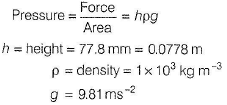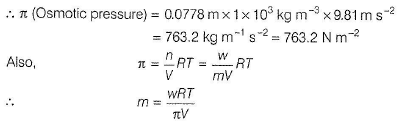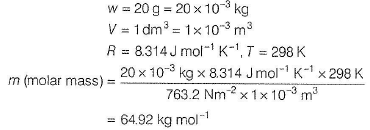Courses

# Test: Colligative Properties IV (Osmotic Pressure)

## 20 Questions MCQ Test Chemistry Class 12 | Test: Colligative Properties IV (Osmotic Pressure)

Description
This mock test of Test: Colligative Properties IV (Osmotic Pressure) for Class 12 helps you for every Class 12 entrance exam. This contains 20 Multiple Choice Questions for Class 12 Test: Colligative Properties IV (Osmotic Pressure) (mcq) to study with solutions a complete question bank. The solved questions answers in this Test: Colligative Properties IV (Osmotic Pressure) quiz give you a good mix of easy questions and tough questions. Class 12 students definitely take this Test: Colligative Properties IV (Osmotic Pressure) exercise for a better result in the exam. You can find other Test: Colligative Properties IV (Osmotic Pressure) extra questions, long questions & short questions for Class 12 on EduRev as well by searching above.
QUESTION: 1

### Only One Option Correct Type This section contains 17 multiple choice questions. Each question has four choices (a), (b), (c) and (d), out of which ONLY ONE is correct Q. Osmosis is involved in one or more processes. I. Excretion of urine II. Reverse osmosis III. Evaporation IV. Interchange of nutrients and waste products between tissue cells and their surroundings. Select the correct processes.

Solution:

I. Excretion of urine.
II. Reverse osmosis is used in desalination of sea water.
IV. Interchange of nutrients and waste products between tissue cells and their surroundings.
These are the processes involving osmosis.

QUESTION: 2

### Osmotic pressure of insulin solution at 298 K is found to be 0.0072 atm at 298 K. Hence, height of water column due to this pressure is (density of mercury = 13.6 g mL-1)

Solution:

(c) Pressure (due to insulin) = 0.0072 atm

= 0.0072 x 76 cm of Hg

= 0.0072 x 76 x 13.6 cm of Hg column

Height of water column = h cm

∴ p = h x 1 cm of water column

(Density of water = 1 g mL-1)

∴ h x 1 = 0.0072 x 76 x 13.6 = 7.44 cm

QUESTION: 3

### Which has maximum osmotic pressure at temperature T K?

Solution: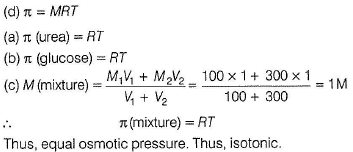QUESTION: 4

There is formation of Prussian blue when Fe3+ reacts with [Fe(CN)6]4-. Two solutions as shown are separated by a semipermeable membrane AB. Due to osmosis, there is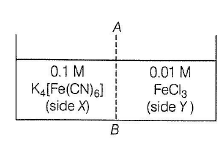Solution:

Side X (0.1 M)            Side Y (0.01 M)

(Concentrated)              (Dilute)

There is osmosis of solvent (H20) from dilute solution (Y) to concentrated solution (X). There is no osmosis of ions, hence no blue colour formation.

QUESTION: 5

Three solutions of following in water are isothermal conditions as given: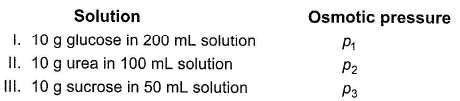Thus,

Solution: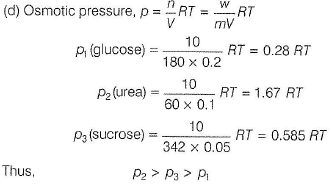QUESTION: 6

What will be the ratio of the masses of formalin (HCHO) and glucose (C6H1206) contained in equal volumes of solutions having the same osmotic pressure at the given temperature?

Solution: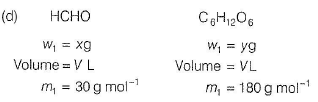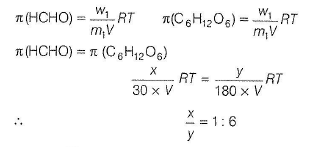QUESTION: 7

A solution containing 0.4 g of a non-volatile solute in 0.1 dm3 of the solution exerts an osmotic pressure of 66.66 kPa at 300 K. Thus, molar mass of the solute is

Solution: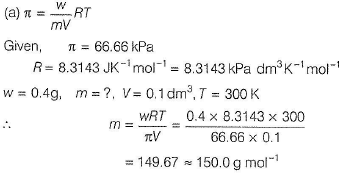QUESTION: 8

For a dilute aqueous solution, density is 1 .00 g cm3 and g (acceleration due to gravity) is 10.0 ms-2. Then, osmotic pressure set up at highest of 0.17 m is

Solution:

(c

At a column of height h.
Osm otic pressure π = pgh = density x g x h Density, ρ = 1g cm-3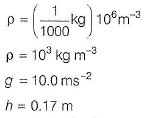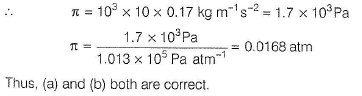QUESTION: 9

Sea water is approximately 0.70 M in NaCI. It can be desalinated by reverse osmosis. For 50% recovery, additional osmotic pressure required (on sea water side) at 298 K is

Solution: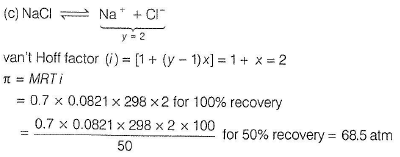QUESTION: 10

A 5% solution of cane sugar (molar mass = 342 g mol-1) is isotonic with 1 % of a solution of an unknown non-electrolyte solute at 300 K. The molar mass of unknown solute is (in g mol-1)   [Aieee 2011]

Solution: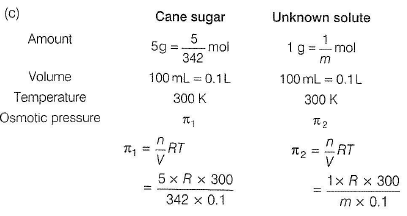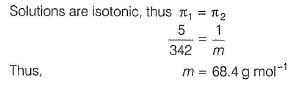QUESTION: 11

5.25% solution of a substance is isotonic with a 21 .5% solution of urea (NH2CONH2 )by weight of solution in the same solvent at 300 K. If the densities of both the solutions are assumed to be equal to 1.0 g cm-3 then, molar mass of the substance will be

Solution: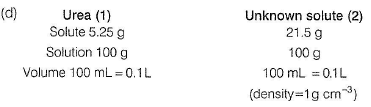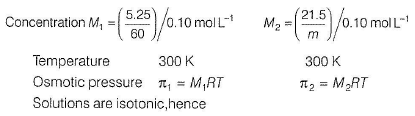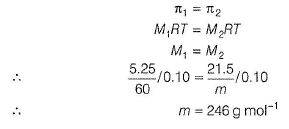QUESTION: 12

Freezing point of a biological fluid is - 0.56° C in aqueous solution.Kf (H20) = 1 .86° mol-1 kg. Thus, its osmotic pressure at 310 K is (assume molarity = molality)

Solution:

ΔTf = molality x Kf

0.56° = molality x 1,86° mol-1 kg

∴ Molarity =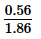mol kg-1
or molarity = 0.301 mol L-1

Also, π = M RT= 0.301 x 0,0821 x 3 1 0 = 7.66 atm

QUESTION: 13

100 mL of a solution containing 0.4 g urea (NH2CONHand 0.9 g of another substance (X ) shows an osmotic pressure of 2.85 atm at 25° C. Molar mass of the substance (X)is

Solution: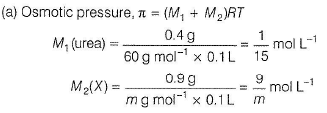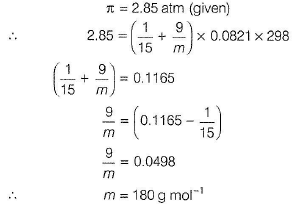QUESTION: 14

A solution containing 1 g of glucose and 1 g of sucrose in 1 L solution is found to be isotonic with a solution containing 2 g of another unknown solute (X) in 1 L solution at 300 K. Thus, molar mass of the solute X is

Solution: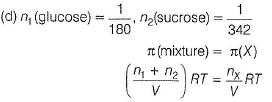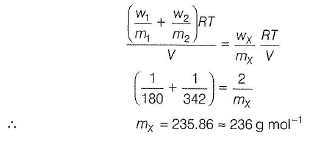QUESTION: 15

The vapour pressure of pure benzene at 353 K is 10.0268 x 104 Nm-2 and that of a solution containing non-volatile solute in benzene is 9.89600 x 104 Nm-2. (d of benzene = 8.149 x 102 g dm-3). Thus, osmotic pressure of the solution is

Solution: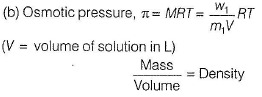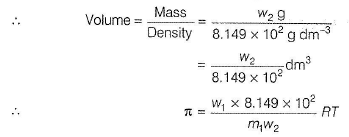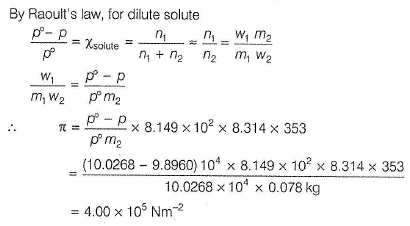QUESTION: 16

Blood plasma has following composition,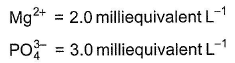and other ions. What is the osmotic pressure set up at 300 K due to Mg2+and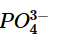ions?

Solution: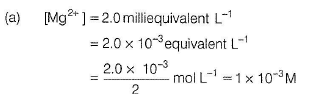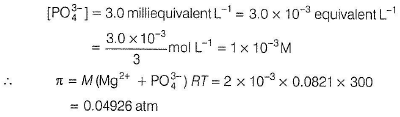QUESTION: 17

Osmotic pressure of 3% aqueous solution of a non-volatile, non-electrolyte solute A at 25° C is 3.632 atm. Thus, its vapour pressure is (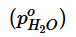at 25° C is 23.7 mm) (assume dilute solution)

Solution: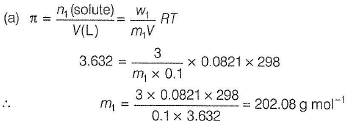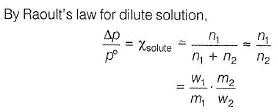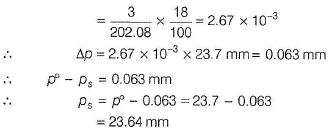QUESTION: 18

Statement Type
This section is based on Statement I and Statement II. Select the correct anser from the codes given below

Q. Statement I  : Osmotic pressure of 34.2% sucrose solution at 300 K is 30.0 atm.
Statement II : π = MRT is valid for dilute solution.

Solution: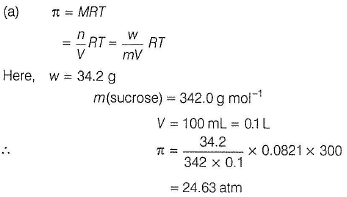But, experimental value is 30.0 atm. This value is much higher than the theoretical value.
It is because (π = mRT) is valid for dilute solution.
Thus, both Statements I and II are correct and Statement II is the correct explanation of Statement I.

QUESTION: 19

Matching List Type
Choices for the correct combination of elements from Column I and Column II are given as options (a), (b), (c) and (d), out of which one is correct

Q. Match the terms in Column I with the corresponding definition in Column II and select the answer from the codes given below.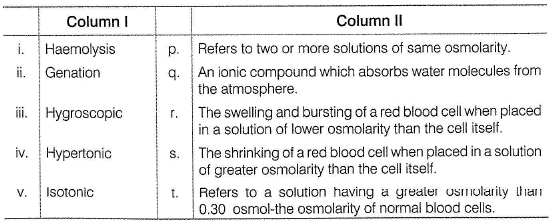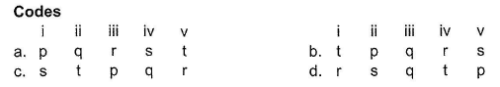Solution:

(d) As per definition

Thus, (i) → (r), (ii) → (s), (iii) → (q), (iv) → (t), (v) → (p)

QUESTION: 20

Comprehension Type
This section contains a passage describing theory, experiments, data, etc. Two questions related to the paragraph have been given. Each question has only one correct answer out of the given 4 options (a), (b), (c) and (d)

Passage

Consider the following arrangement in which a solution containing 20 g of haemoglobin in 1 L of the solution is placed in the right compartment and pure water is placed in the left compartment. At equilibrium, the height of the water in the right column is 77.8 mm in excess of the height of the solution in the left column at 298 K.

Q. Osmotic pressure of the solution is

Solution: